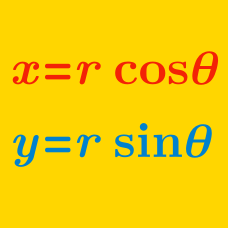Algebra

# Polar Coordinates Warmup

The point $P$ has Cartesian coordinates $(3, 4)$ and polar coordinates $(r, \theta).$ What is the value of $r$?

The point $P$ has Cartesian coordinates $(0, 1)$ and polar coordinates $(r, \theta).$ What is the value of $\theta$ (in radians)?

What are the polar coordinates of the point whose Cartesian coordinates are $(0, 1)?$

The point $P$ has polar coordinates $(1, 0).$ The answer choices are the polar coordinates of various points - which one is also at $P?$

What are the polar coordinates of the point having Cartesian coordinates $(5, 0)?$

×